# Coulombs Law p 538 in your book Two

• Slides: 13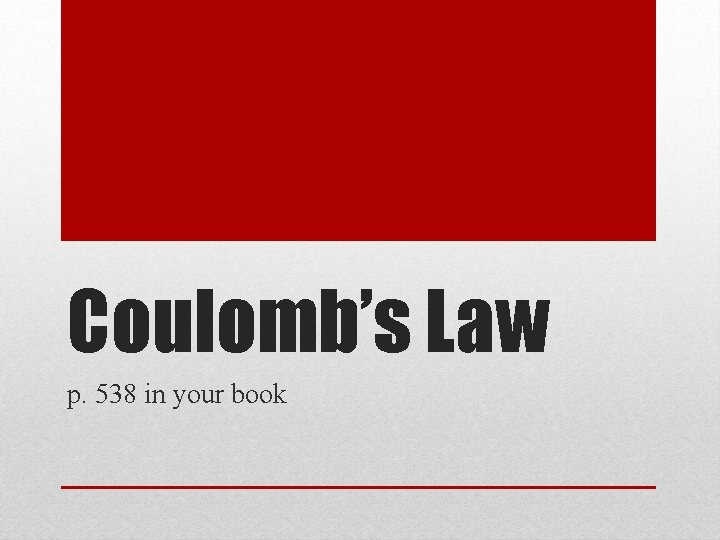Coulomb’s Law p. 538 in your book• Two electrically charged objects exert a force on each other. • Opposite charges ATTRACT • Notice the forces are “equal but opposite” Charged objects & electrical force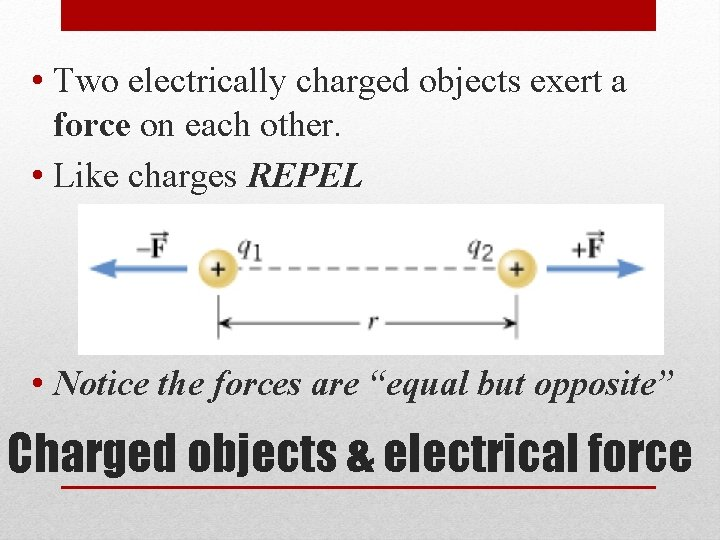• Two electrically charged objects exert a force on each other. • Like charges REPEL • Notice the forces are “equal but opposite” Charged objects & electrical force• Coulomb’s Law• Coulomb’s Law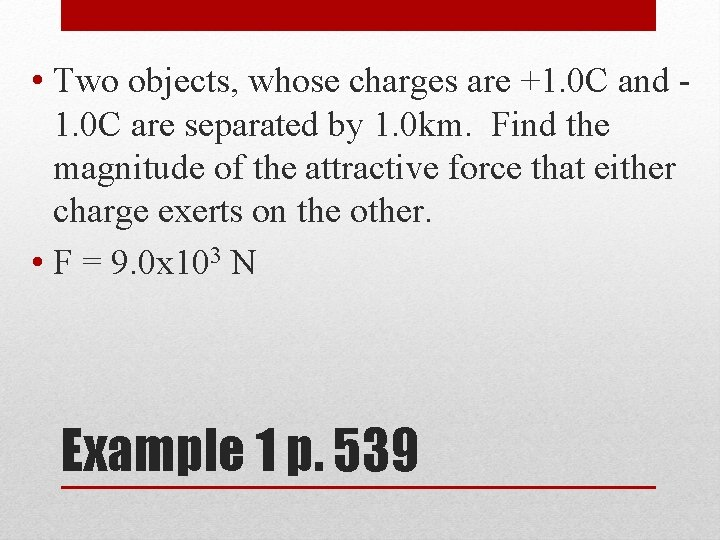• Two objects, whose charges are +1. 0 C and 1. 0 C are separated by 1. 0 km. Find the magnitude of the attractive force that either charge exerts on the other. • F = 9. 0 x 103 N Example 1 p. 539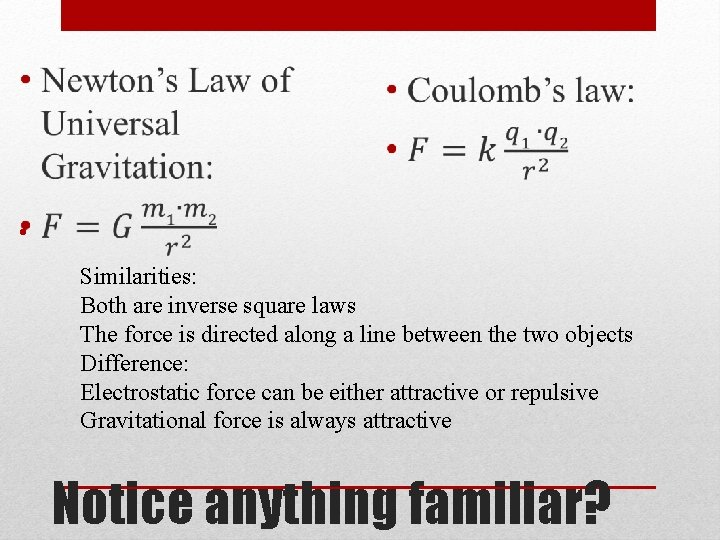• Similarities: Both are inverse square laws The force is directed along a line between the two objects Difference: Electrostatic force can be either attractive or repulsive Gravitational force is always attractive Notice anything familiar?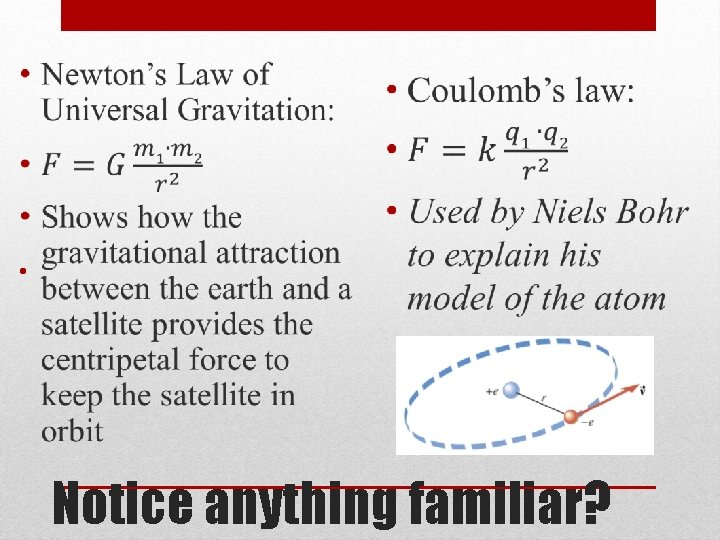• Notice anything familiar?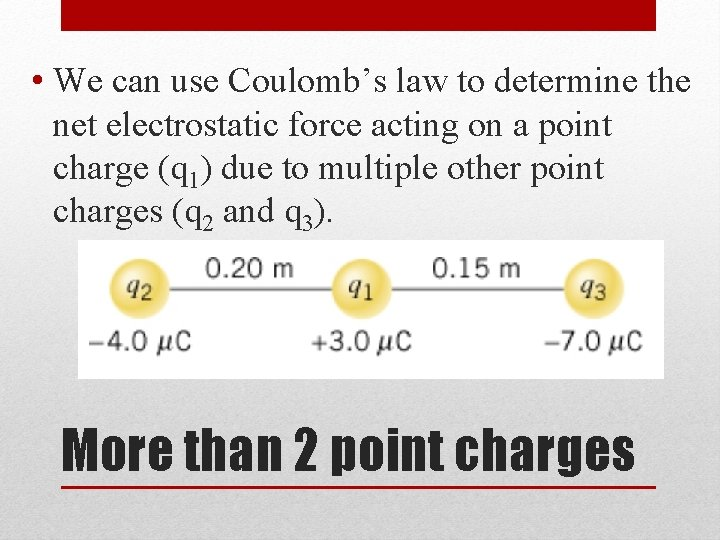• We can use Coulomb’s law to determine the net electrostatic force acting on a point charge (q 1) due to multiple other point charges (q 2 and q 3). More than 2 point charges• First determine the force (magnitude and direction!) that q 3 exerts on q 1 (ignore q 2) • Then determine the force (magnitude and direction!) that q 2 exerts on q 1 (ignore q 3) More than 2 point charges• The net force on q 1 is the vector sum of these two forces. More than 2 point charges• Determine the magnitude and direction of the net electrostatic force on q 1. • +5. 7 N Example 4 p. 541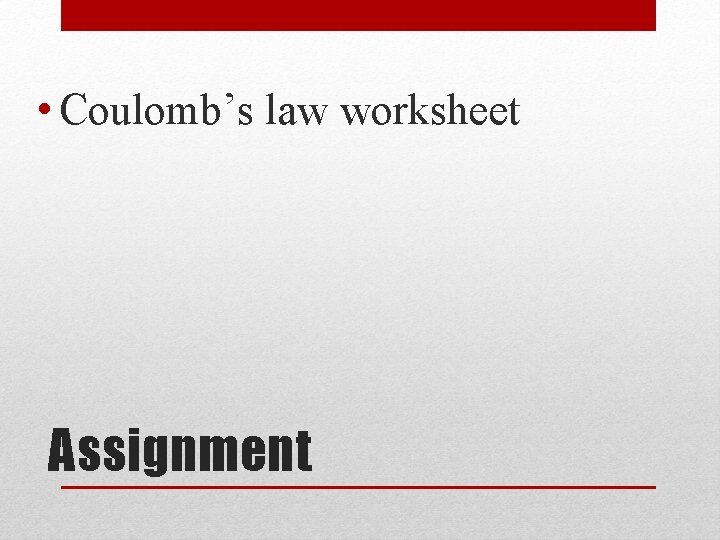• Coulomb’s law worksheet Assignment